## General Question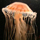# Calculate the best estimate for the conductance G?

Asked by againto (7) December 26th, 2009

A student is measuring the conductance of a component.
He measures the current in the component as I = 0.24 + 0.01 A
and the p.d. across the component as V = 5.4 + 0.1 V.

Calculate the best estimate for the conductance G

I’m not sure how you’re suppose to work this out, could you explain what they mean and how to work it out?

also.

State an estimated value for the + uncertainty in the measurement of G.

Observing members: 0Composing members: 0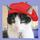Could the student ask the teacher?

gailcalled (54644)“Great Answer” (0) Flag as…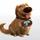Regarding this question how are you thinking of going about it?

For homework problems we do not mind offering gentle help or a point in the right direction to help you find the solution but you need to let us know what direction you are taking to attempt to solve the problem.

Dog (25152)“Great Answer” (0) Flag as…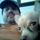Conductance is the inverse of resistance
I would calculate the resitance of the component
I assume the + 0.01 amp and +.1 V are the uncertainties?
You need to calculate at both minimum and maximum to get the uncertainty
Or look Here

csimme01 (550)“Great Answer” (0) Flag as…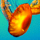Remember that your units will be in mhos (inverse of ohms). @csimme01 reference will get you there, including the statistical analysis for the uncertainties. Remember your significant digits, where many students fall down. Just because your calculator will give a number with 12 digits it doesn’t mean they are worth a shit beyond your least certain measurement.One of the advantages of being a dinosaur who can still use a sliderule.

stranger_in_a_strange_land (18355)“Great Answer” (0) Flag as…or